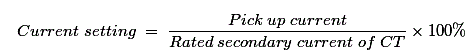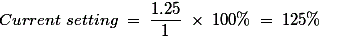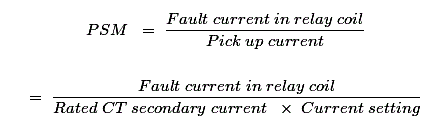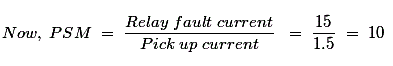# Pick Up Current | Current Setting | Plug Setting Multiplier and Time Setting Multiplier of Relay

During study of electrical protective relays, some special terms are frequently used. For proper understanding, the functions of different protective relays, the definition of such terms must be understood properly. Such terms are,

1. Pick up current.
2. Current setting.
3. Plug setting multiplier (PSM).
4. Time setting multiplier (TSM).

## Pick Up Current of Relay

In all electrical relays, the moving contacts are not free to move. All the contacts remain in their respective normal position by some force applied to them continuously. This force is called the controlling force of the relay. This controlling force may be the gravitational force, may be a spring force, or may be a magnetic force.
The force applied to the relay’s moving parts for changing the normal position of the contacts is called deflecting force. This deflecting force is always in opposition of controlling force and is present always in the relay. Although the deflecting force always presents in the relay directly connected to live line, as the magnitude of this force is less than controlling force in normal condition, the relay does not operate. If the actuating current in the relay coil increases gradually, the deflecting force in electromechanical relay is also increased. Once, the deflecting force crosses the controlling force, the moving parts of the relay initiate to move to change the position of the contacts in the relay. The current for which the relay initiates its operation is called pick up current of relay.

## Current Setting of Relay

The minimum pick up the value of the deflecting force of an electrical relay is constant. Again the deflecting force of the coil is proportional to its number of turns and the current flowing through the coil.
Now, if we can change the number of active turns of any coil, the required current to reach at minimum pick value of the deflecting force, in the coil also changes. That means if active turns of the relay coil are reduced, then proportionately more current is required to produce desired relay actuating force. Similarly, if active turns of the relay coil are increased, then proportionately reduced current is required to produce same desired deflecting force.

Practically same model relays may be used in different systems. As per these system requirements, the pickup current of the relay is adjusted. This is known as the current setting of the relay. This is achieved by providing the required number of tapping in the coil. These taps are brought out to a plug bridge. The number of active turns in the coil can be changed by inserting a plug in different points in the bridge.
The current setting of relay is expressed in percentage ratio of relay pick up current to the rated secondary current of CT.

That means,For example, suppose, you want that, an over current relay should operate when the system current just crosses 125% of rated current. If the relay is rated with 1 A, the normal pick up current of the relay is 1 A and it should be equal to secondary rated current of current transformer connected to the relay.
Then, the relay will be operated when the current of CT secondary becomes more than or equal to 1.25 A.
As per definition,The current setting is sometimes referred as current plug setting.
The current setting of overcurrent relay is generally ranged from 50 % to 200 %, in steps of 25 %. For earth fault relay it is from 10% to 70% in steps of 10%.

## Plug Setting Multiplier of Relay

Plug setting multiplier of relay is referred as ratio of fault current in the relay to its pick up current.Suppose we have connected on protection CT of ratio 200/1 A and current setting is 150%.
Hence, pick up current of the relay is, 1 × 150 % = 1.5 A
Now, suppose fault current in the CT primary is 1000 A. Hence, fault current in the CT secondary, i.e., in the relay coil is, 1000 × 1/200 = 5A
Therefore PSM of the relay is, 5 / 1.5 =3.33

## Time Setting Multiplier of Relay

The operating time of an electrical relay mainly depends upon two factors :

1. How long distance to be traveled by the moving parts of the relay for closing relay contacts and
2. How fast the moving parts of the relay cover this distance.

So far by adjusting relay operating time, both of the factors to be adjusted. The adjustment of traveling distance of an electromechanical relay is commonly known as time setting. This adjustment is commonly known as time setting multiplier of relay. The time setting dial is calibrated from 0 to 1 in steps of 0.05 sec.
But by adjusting only time setting multiplier, we cannot set the actual time of operation of an electrical relay. As we already said, the time of operation also depends upon the speed of operation. The speed of moving parts of relay depends upon the force due to the current in the relay coil. Hence, it is clear that the speed of operation of an electrical relay depends upon the level of fault current. In other words, the time of operation of the relay depends upon plug setting multiplier. The relation between time of operation and plug setting multiplier is plotted on a graph paper, and this is known as time/PSM graph. From this graph one can determine the total time taken by the moving parts of an electromechanical relay, to complete its total traveling distance for different PSM. In time setting multiplier, this total traveling distance is divided and calibrated from 0 to 1 in steps of 0.05.
So when the time setting is 0.1, the moving parts of the relay have to travel only 0.1 times of the total traveling distance, to close the contact of the relay. So, if we get the total operating time of the relay for a particular PSM from time/PSM graph and if we multiply that time with the time setting multiplier, we will get, the actual time of operation of the relay for said PSM and TSM.
For getting a clear idea, let us have a practical example. Say a relay has a time setting 0.1 and you have to calculate actual time of operation for PSM 10.
From the time / PSM graph of the relay as shown below, we can see the total operating time of the relay is 3 seconds. That means the moving parts of the relay take total 3 seconds to travel 100 % traveling distance. As the time setting multiplier is 0.1 here, actually the moving parts of the relay have to travel only 0.1 × 100% or 10% of the total travel distance, to close the relay contacts.
Hence, actual operating time of the relay is 3 × 0.1 = 0.3 sec. i.e. 10% of 3 sec.

## Time vs. PSM Curve of Relay

This is relation curve between operating time and plug setting multiplier of an electrical relay. The x-axis or horizontal axis of the Time/PSM graph represents PSM and Y-axis, or vertical axis represents the time of operation of the relay. The time of operation represents in this graph is that, which required to operate the relay when time setting multiplier set at 1.
From the Time/PSM curve of a typical relay shown below, it is seen that, if PSM is 10, the time of operation of the relay is 3 sec. That means the relay will take 3 seconds to complete its operation, with the time setting 1.
It is also seen from the curve that, for a lower value of plug setting multiplier, i.e., for a lower value of fault current, the time of operation of the relay is inversely proportional to the fault current.
But when PSM becomes more than 20, the operating time of relay becomes almost constant. This feature is necessary to ensure discrimination on very heavy fault current flowing through sound feeders.

## Calculation of Relay Operation Time

For calculating actual relay operating time, we need to know these following operations.

1. Current setting.
2. Fault current level.
3. Ratio of current transformer.
4. Time / PSM curve.
5. Time setting.

Step-1
From CT ratio, we first see the rated secondary current of CT. Say the CT ratio is 100 / 1 A, i.e. secondary current of CT is 1 A.

Step-2
From current setting we calculate the trick current of the relay. Say current setting of the relay is 150 % therefore pick up current of the relay is 1 × 150% = 1.5 A.

Step-3
Now we have to calculate PSM for the specified faulty current level. For that, we have to first divide primary faulty current by CT ratio to get relay faulty current. Say the faulty current level is 1500 A, in the CT primary, hence secondary equivalent of faulty current is 1500/(100/1) = 15 AStep-4
Now, after calculating PSM, we have to find out the total time of operation of the relay from Time/PSM curve. From the curve, say we found the time of operation of relay is 3 second for PSM = 10.

Step-5
Finally that operating time of relay would be multiplied with time setting multiplier, in order to get actual time of operation of relay. Hence say time setting of the relay is 0.1.
Therefore the actual time of operation of the relay for PSM 10, is 3 × 0.1 = 0.3 sec or 300 ms.

Want To Learn Faster? 🎓
Get electrical articles delivered to your inbox every week.
No credit card required—it’s 100% free.